# Magnetic behavior of materials: definitions

## Definition

The macroscopic dependence linking the local values of the vector quantities magnetization M (or magnetic polarization J ) and the magnetic field strength H in any point of a material is called the law of material magnetic behavior.

This law renders the fact that the magnetization of matter is modified under the effect of a magnetic field.

This law is written in the form:

•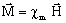with the magnetization vector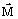•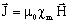with the polarization vector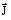where χm is the scalar or the tensor of magnetic susceptibility*

Attention: * the quantity χm depends on H; χm should be written in the form χm(H) in all the relationships above.

## Notation

The magnetization law is written in the form: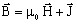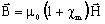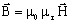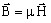where μ is the scalar or the tensor of permeability

Attention: * the quantities χm, μr, μ should be written in the form χm(H), μr(H), μ(H) in all the relationships above.

## Complex permeability

In the case of harmonic applications, a complex representation of permeability is used.

The quantity μ can be expressed by the complex permeability:

μ = μ' - j μ"

where:

• μ' corresponds to the real part of the complex permeability
• μ" corresponds to the imaginary part of the complex permeability